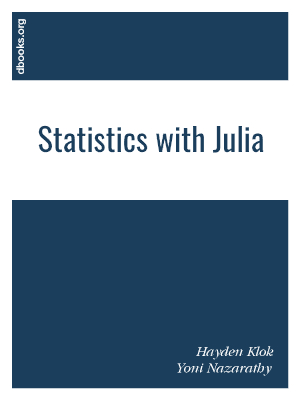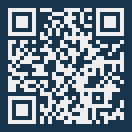# Statistics with Julia

## Fundamentals for Data Science, Machine Learning and Artificial Intelligence

by Hayden Klok, Yoni Nazarathy### Book Description

Ccurrently many of Julia's users are hard-core developers that contribute to the language's standard libraries, and to the extensive package eco-system that surrounds it. Therefore, much of the Julia material available at present is aimed at other developers rather than end users. This is where our book comes in, as it has been written with the end-user in mind. The code examples have been deliberately written in a simple format, sometimes at the expense of efficiency and generality, but with the advantage of being easily readable. Each of the code examples aims to convey a specific statistical point, while covering Julia programming concepts in parallel. In a way, the code examples are reminiscent of examples that a lecturer may use in a lecture to illustrate concepts. The content of the book is written in a manner that does not assume any prior statistical knowledge, and in fact only assumes some basic programming experience and a basic understanding of mathematical notation.

Chapter 1
Introducing Julia
Chapter 2
Basic Probability
Chapter 3
Probability Distributions
Chapter 4
Processing and Summarizing Data
Chapter 5
Statistical Inference Ideas
Chapter 6
Confidence Intervals
Chapter 7
Hypothesis Testing
Chapter 8
Linear Regression
Chapter 9
Machine Learning Basics
Chapter 10
Simulation of Dynamic Models
Appendix A
How-to in Julia
Appendix B
Appendix C

Subject
Computer Science
Publisher
Self-publishing
Published
2020
Pages
413
Edition
1
Language
English
PDF Size
13.3 MB

### Related BooksThis ICME-13 Topical Survey provides a review of recent research into statistics education, with a focus on empirical research published in established educational journals and on the proceedings of important conferences on statistics education. It identifies and addresses six key research topics, namely: teachers' knowledge; teachers' role in stat...If you know how to program with Python and also know a little about probability, you're ready to tackle Bayesian statistics. With this book, you'll learn how to solve statistical problems with Python code instead of mathematical notation, and use discrete probability distributions instead of continuous mathematics. Once you get the math out of the ...The demand for skilled data science practitioners in industry, academia, and government is rapidly growing. This book introduces concepts and skills that can help you tackle real-world data analysis challenges. It covers concepts from probability, statistical inference, linear regression and machine learning. It also helps you develop skills such a...This open access textbook provides the background needed to correctly use, interpret and understand statistics and statistical data in diverse settings. Part I makes key concepts in statistics readily clear. Parts I and II give an overview of the most common tests (t-test, ANOVA, correlations) and work out their statistical principles. Part III pr...The revised 2nd edition of this book provides the reader with a solid foundation in probability theory and statistics as applied to the physical sciences, engineering and related fields. It covers a broad range of numerical and analytical methods that are essential for the correct analysis of scientific data, including probability theory, distribut...Introductory Business Statistics is designed to meet the scope and sequence requirements of the one-semester statistics course for business, economics, and related majors. Core statistical concepts and skills have been augmented with practical business examples, scenarios, and exercises. The result is a meaningful understanding of the discipline, w...